+

Author: Sophia Tutorial
##### Description:

Identify a parabola given a vertex and solution set.

(more)

Sophia’s self-paced online courses are a great way to save time and money as you earn credits eligible for transfer to many different colleges and universities.*

No credit card required

37 Sophia partners guarantee credit transfer.

299 Institutions have accepted or given pre-approval for credit transfer.

* The American Council on Education's College Credit Recommendation Service (ACE Credit®) has evaluated and recommended college credit for 32 of Sophia’s online courses. Many different colleges and universities consider ACE CREDIT recommendations in determining the applicability to their course and degree programs.

Tutorial
what's covered
1. Quadratics as Second Degree Polynomials
3. Parabolas

# 1. Quadratics as Second Degree Polynomials

A quadratic is a second degree polynomial, which means that in the expression of a quadratic, there will be no more than 2 x-terms being multiplied together. In expanded form, the highest exponent you will see is 2, and in factored form, there will only be two factors of x. For example: (x+3)(x–2) is a quadratic, but (x+3)(x–2)(x+1) is not, because there are 3 factors of x.

term to know
A second degree polynomial, with an x-squared term as its highest degree term.

# 2. Forms of Quadratic Equations

There are several different ways to write a quadratic equation. We are going to cover standard form, vertex form, and factored form:

formula
Standard Form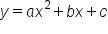This is the expanded form of quadratic expressions. There is an x-squared term, an x-term, and a constant term. We use the coefficients a, b, and c, which are the same coefficients used to solve quadratic equations using the quadratic formula.

formula
Vertex Form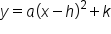Vertex form is ideal for graphing quadratic equations, because it provides information about the parabola's vertex readily in its equation. The variables h and k represent the x– and y–coordinates to the vertex. The vertex is the point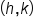.

formula
Factored Form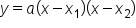Equations in factored form allow us to easily identify the x-intercepts of the parabola, which we will later discuss as solutions to the quadratic equation.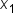and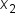represent x-values at which y is equal to zero.

# 3. Parabolas

When quadratic equations are graphed, we call the curve a parabola. It has a distinct U shape to it (or an upside down U shape if the parabola opens downward). There is also either a minimum or a maximum point (also dependent upon which direction the parabola opens). This maximum or minimum point is known as the vertex of the parabola, and it lies on a line of symmetry to the graph. This means that one half of the parabola can be reflected about that line of symmetry to match up perfectly with the other half of the parabola.

term to know
Parabola
The shape of a quadratic equation on a graph; it is symmetric at the vertex.
Vertex (of a parabola)
The maximum or minimum point of a parabola located on the axis of symmetry.

Here are a few graphs of parabolas. See if you can spot the vertex, and notice its symmetry: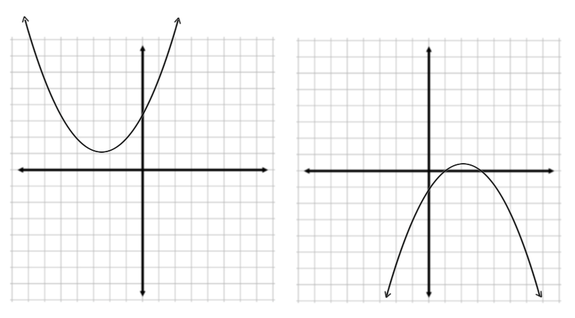hint
The leading coefficient to the equation (the value of "a" in each of the three forms above) determine whether the parabola opens up or down. If a is positive, the parabola opens up (and has a U shape). If a is negative, the parabola opens down (and has an upside down U shape).

# 4. Solutions to Quadratic Equations

A solution to a quadratic equation is also referred to as a zero, or a root. This is because solutions are x-values that make y equal to zero. Graphically, these are x-intercepts to the parabola. Quadratic equations can have zero, one, or two real solutions. There will never be three real solutions to the equation. This is because parabolas can intersect the x-axis at most 2 times.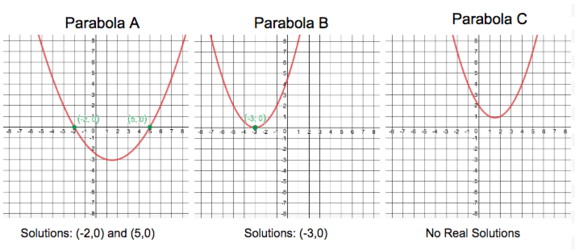There are several different methods to solving a quadratic equation. The most common ways are by using the Zero Factor Property, and using the Quadratic Formula. These methods are covered in greater detail elsewhere, but in general:

• The Zero Factor Property takes advantage of the fact that anything multiplied by zero equals zero. We set each factor of the quadratic equal to zero and solve for x. This is the ideal method when working with equations written in factored form.
• The Quadratic Formula uses the coefficients a, b, and c from equations written in standard form, and set equal to zero. Plugging in these values, and performing the algebraic steps to solve for x will give solutions to the quadratic that might not be easily solved by factoring.
summary
When we think of quadratics, we can think of quadratics as second degree polynomials. There are three different forms of quadratic equations: standard, vertex, and factored. The graph of a quadratic equation is called a parabola. Parabolas have either a minimum or a maximum point, which is called the vertex. The solutions to quadratic equations can also be called a root or a 0. The solution represents the x-intercepts of the parabola on a graph. And a quadratic equation can be solved algebraically by substituting 0 for y in the equation, and solving for x.
Terms to Know
Parabola

the shape of a quadratic equation on a graph; it is symmetric at the vertex

a second degree polynomial, with an x-squared term as its highest degree term

Vertex (of a parabola)

the maximum or minimum point of a parabola located on the axis of symmetry

Formulas to Know
Factored Form of a Quadratic Equation